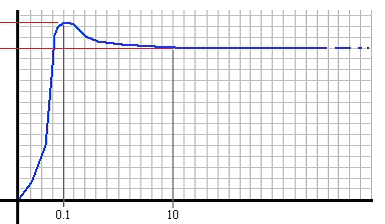# Friction Overview

Friction, a resistive force that occurs in joints and between parts in contact, is calculated based on the static and dynamic coefficients of friction and the normal force acting on the part.

Mate friction is more complex because the size of the contact area can affect the magnitude of friction.

## Mate Friction

You can specify joint friction on the Analysis tab of the Mate PropertyManager. It is a resistive, sliding, surface force between parts that must be overcome for the parts to move with respect to one another. The force develops due to contact between the surfaces and the loads acting on the connection. Joint friction takes into account standard friction between bodies as well as aspects of joint geometry in determining the net frictional forces acting.

The SOLIDWORKS Motion mate friction model uses a combination of dimensional information and a coefficient of friction. You can enter the coefficient of friction directly, or you can have SOLIDWORKS Motion calculate it based on your choice of materials.

Mate friction is modeled as Coulomb friction and only represents the dynamic friction in the joint.

## Contact Friction

You can assign contact friction (friction that occurs between bodies in contact) in the Contact PropertyManager. The velocities and coefficients of friction used are assigned automatically, based on the materials you define for each contact set. However, they may not be the most appropriate parameters based on the dynamics of the model. You can set these coefficients manually, if necessary.

SOLIDWORKS Motion uses the Coulomb friction method and fits a smooth curve to the friction parameters to solve for the friction force.

For this example, the default friction parameters for dry steel in contact with dry steel are used.

Dynamic Friction Transition Velocity: 10.16 mm/s

Dynamic Friction Coefficient: 0.25

Static Friction Transition Velocity: 0.1 mm/s

Static Friction Coefficient: 0.30

 Frictional Force Static DynamicForce (N) Velocity (mm/sec)

In the Coulomb friction method, friction forces are calculated based on four constants:
 Dynamic Friction Velocity The velocity at which dynamic friction becomes constant. Dynamic Friction Coefficient The constant used to calculate friction forces once the body is no longer at rest. Static Friction Velocity: The velocity at which transition occurs between a stationary component and a moving component. Static Friction Velocity The velocity at which transition occurs between a stationary component and a moving component. Static Friction Coefficient The constant used to calculate the force necessary to overcome friction when a body is at rest.
To edit the velocity and coefficient parameters, clear Material in the Contact PropertyManager.